# Random date in Excel

Generate random date in Excel is easy. Let’s see how to do that.

## Date as a number

Each date in a cell is always represented by number. The cell formatting does that you see it as a date. So, you can use RANDBETWEEN function.

## RANDBETWEEN

Function RANDBETWEEN generates a random integer number between the numbers you specify.

=RANDBETWEEN(startNumber,endNumber)

You can place cell address with a date instead of startNumber and endNumber (example 1). Or you can write date number right into the formula (example 2). To find the startNumber and endNumber just change cell format from date to number.

Note: The random date will change every time you update the worksheet.

## Example 1

Task: Generate random date between 01/01/2013 and 12/31/2013.

1. Write both dates into cells.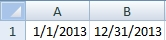2. Insert =RANDBETWEEN(A1,B1) into a cell. (41422 is a random number, probably will be always different)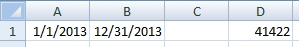3. Format cell as a date.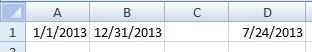## Example 2

The same task as in the previous example: Generate random date between 01/01/2013 and 12/31/2013.

1. Write both dates into cells and change format to number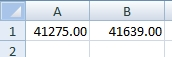2. Insert =RANDBETWEEN(41275,41639) into a cell. (The cells A1 and B1 are no longer needed.)
3. Format cell as a date.

Need generate random dates with more specific parameters? Try Online Random Date Generator

1.What about random date with special format? Does anybody know the solution?

2.Hi,

I need generate random weekends only. Is it possible to do that without macro? I am a beginner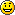3.I used function RANDBETWEEN and it works. I have 2 dates and I generate multiple random dates between them. I want to exclude some weekdays. How can I do it?